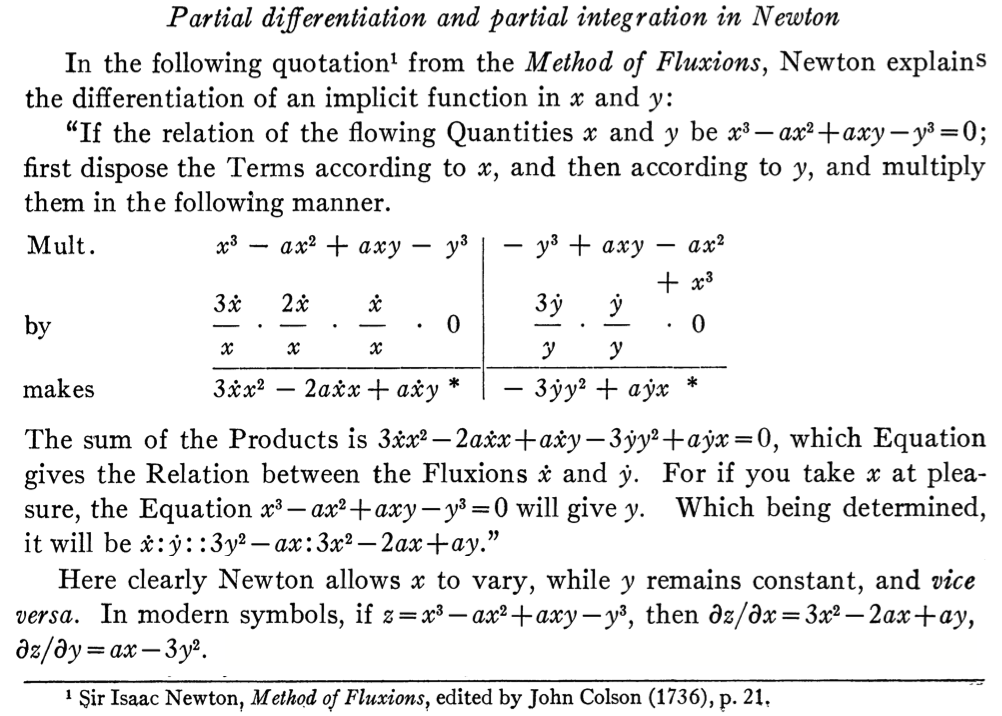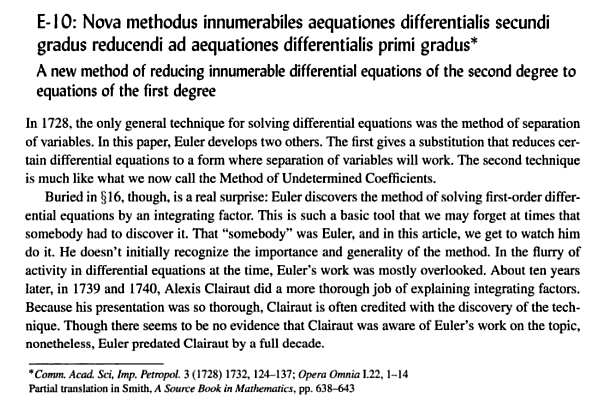# history of calculus of several variables

Everybody knows that Leibniz and Newton (or Newton and Leibniz, if you wish) invented calculus, i.e. they developed the notion of differentiability for a function of one real variable. But who had for the first time the right idea about generalizing their notions to functions of several variables? I am talking in particular about total differentiability.

According to Ref. 1, see first screenshot below, Newton already had partial differentiation in his Method of Fluxions (1671). (Whether or not he also introduced partial differential equations is a matter of debate.)

According to Ref. 2, see second screenshot, the notion of an integrating factor goes back to Euler (1728), followed a decade later by Clairaut's more systematic treatment of total differentials.

1. Florian Cajori, The Early History of Partial Differential Equations and of Partial Differentiation and Integration
2. C. Edward Sandifer, The Early Mathematics of Leonhard Euler

From Ref. 1, page 463:From Ref. 2, page 22:• Euler's Institutiones calculi differentialis is not from 1728 but from 1755. The integrability condition (existence of integrating factor) goes back earlier than that: according to Euler's Opera Omnia, vol. 4A, p. 87,... Jan 30, 2014 at 1:42
• ... the integrability condition "is stated in Clairaut [1742, p. 307]. Euler uses it in [1750, p. 197], attributing it to Clairaut and d'Alembert. In , d'Alembert formally declared that this theorem does not belong to him but to Fontaine." Jan 30, 2014 at 1:42
• than you, @FrancoisZiegler, for the expert corrections/expansions. I have corrected the 1728 source (it was another one of Euler's publications) and added a link to a discussion of Euler versus Clairaut. Jan 30, 2014 at 13:08
• Thanks Carlo. I found your ref. 2 fully available here -- and must say I'm yet unconvinced of its relevance to 'calculus of several variables'. Jan 30, 2014 at 16:36
• Sandifer is also wrong to say that Euler (1728, §16) discovers integrating factors when he multiplies $\frac{dz}{dt}+pz+q=0$ by $\mu=e^{\int\!p\,dt}$ to get $\frac d{dt}(\mu z)+\mu q=0$ with solution $\mu z+\int \mu q\,dt =\text{const.}$ (with $p=\frac2{t-1}$ and $q=\frac1{t^2-t}$): that was first done by Leibniz (1694, p. 257; 1696) and advertised by Bernoulli (1697) (make $n=0$). Oct 27, 2018 at 21:06

Functions of several variables in the modern sense were never systematically considered in the 17th century. Instead the idea of partial differentiation arose in connection with families of curves, through the idea of differentiating with respect to the parameter (as one does for example when finding the envelope of a family of curves). For a synopsis of how this idea arose in the 1697 correspondence between Leibniz and Johann Bernoulli, see my review of Engelsman's Families of Curves and the Origins of Partial Differentiation (1984), an excellent study. Newton never discussed partial derivatives in any systematic way.

• Interesting. When did then functions of - say - two spacial variables begin to be studied? I would guess that this must have been common at latest at the time of D'Alembert (PDEs with physical motivation etc.), but perhaps you have a sharper estimate? Jan 30, 2014 at 17:25

To Carlo Beenakker's references about total differentials (which came first), one might add a word about Alexis Fontaine, inventor of the notation (notion?) of partial derivative:

1. Fontaine, Alexis Le calcul intégral. Première méthode (19 Nov. 1738), in Mémoires donnés à l'Académie royale des sciences, non imprimés dans leur temps. Imprimerie Royale, Paris, 1764. Page 25:

Let $$\mu$$ be a function of several quantities $$p, x, y, z,$$ &c. To denote the coefficient of $$dx$$ in the difference of $$\mu$$, I'll write $$\frac{d\mu}{dx}$$; to denote that of $$dy$$, I'll write $$\frac{d\mu}{dy}$$, &c. By the same reason (...) I'll write $$\frac{dd\mu}{dxdy}$$ or $$\frac{dd\mu}{dydx}$$; for, as we shall soon demonstrate, these two expressions are one and the same thing

N.B.: Although not published until 1764, Fontaine’s memoir was discussed in correspondence of Euler with Clairaut (17 Sep, 19 Oct, 26 Dec 1740) and D. Bernoulli (7 Mar & 16 May 1739).

2. Euler, Leonhard Principia motus fluidorum (31 Aug 1752). Novi Comm. Acad. Sci. Petrop. 6 (1761) 271-311. Page 276:

This system of notation was first used by the illustrious Fontaine; as it appreciably simplifies calculation, I will also adopt it here.

3. Kline, Morris Mathematical thought from ancient to modern times. Oxford University Press, 1972. Page 425:

it was Alexis Fontaine des Bertins (1705-71), Euler, Clairaut, and d'Alembert who created the theory of partial derivatives.

4. Greenberg, John L. Alexis Fontaine's "fluxio-differential method'' and the origins of the calculus of several variables. Ann. of Sci. 38 (1981), no. 3, 251–290. Page 253:

In the years 1738-1741 Fontaine produced an exposition of the calculus of several variables which we recognize to have been remarkably general for those years. (...) Fontaine prefaced his exposition by four elementary but fundamental results in the calculus of several variables: $$\frac{d}{dx}\int F\,dy=\int\frac{dF}{dx}dy$$ (the so-called Leibnizian 'differentiation under the integral sign'); $$\frac{d^2F}{dxdy}=\frac{d^2F}{dydx}$$ (the equality of mixed second-order partial differential coefficients); $$nF=\frac{dF}{dx}x+\frac{dF}{dy}y+\frac{dF}{dz}z+\dots$$ if $$F=F(x,y,z,\dots)$$ is a homogeneous expression of degree $$n$$ in a finite number of variables $$x, y, z, \dots$$; and $$\alpha\frac{d\pi}{dx}-\pi\frac{d\alpha}{dx}+\frac{d\alpha}{dp}-\frac{d\pi}{dy}=0,$$ which is the 'equation of condition' for the integrability of a total differential $$dx+\alpha dy+\pi dp$$ in three variables $$x,y$$ and $$p$$.

[Note: A few pages later Fontaine writes the general case just as we now would: the differential $$Ldp+Mdx+Ndy$$ becomes total when multiplied by an integrating factor only if $$L\Bigl(\frac{dM}{dy}-\frac{dN}{dx}\Bigr)+ M\Bigl(\frac{dN}{dp}-\frac{dL}{dy}\Bigr)+ N\Bigl(\frac{dL}{dx}-\frac{dM}{dp}\Bigr)=0.]$$

5. Greenberg, John L. Alexis Fontaine's integration of ordinary differential equations and the origins of the calculus of several variables. Ann. of Sci. 39 (1982), no. 1, 1–36. Page 10:

Fontaine was among those who pioneered the use of (...) total differentials, while the introduction of whole symbol notations $$\frac{dF}{dx}, \frac{dF}{dy}, \frac{d^2F}{dx^2}, \frac{d^2F}{dxdy}, \frac{d^3F}{dy^3},\dots$$ for differential coefficients (...) was his innovation. As a means of defining partial differential coefficients, the total differential was the earliest development in a recognizable calculus of several variables.

6. Greenberg, John L. The Origins of Partial Differentiation. Ann. of Sci. 42 (1985), no. 4, 421-429. Page 428:

Euler did not invent the 'whole symbol' notation $$\left(\frac{dy}{dx}\right)$$ for partial differential coefficients. The notation appeared in print for the first time in Clairaut's published Paris Academy of Sciences mémoires for 1739 and 1740 on the problems that Fontaine had raised. By 1752, Euler knew that Fontaine had first introduced the notation.